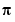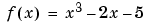MATLAB Function Referencefzero

Find zero of a function of one variable

Syntax

• ```x = fzero(fun,x0)
x = fzero(fun,x0,options)
[x,fval] = fzero(...)
[x,fval,exitflag] = fzero(...)
[x,fval,exitflag,output] = fzero(...)
```

Description

```x = fzero(fun,x0) ``` tries to find a zero of `fun` near `x0`, if `x0` is a scalar. `fun` is a function handle. See Function Handles in the MATLAB Programming documentation for more information. The value `x` returned by `fzero` is near a point where `fun` changes sign, or `NaN` if the search fails. In this case, the search terminates when the search interval is expanded until an `Inf`, `NaN`, or complex value is found.

Parameterizing Functions Called by Function Functions, in the MATLAB mathematics documentation, explains how to provide additional parameters to the function `fun`, if necessary.

If `x0` is a vector of length two, `fzero` assumes `x0` is an interval where the sign of `fun(x0(1))` differs from the sign of `fun(x0(2))`. An error occurs if this is not true. Calling `fzero` with such an interval guarantees `fzero` will return a value near a point where `fun` changes sign.

```x = fzero(fun,x0,options) ``` minimizes with the optimization parameters specified in the structure `options`. You can define these parameters using the `optimset` function. `fzero` uses these `options` structure fields:

 `Display` Level of display. `'off'` displays no output; `'iter'` displays output at each iteration; `'final'` displays just the final output; `'notify'` (default) displays output only if the function does not converge. FunValCheck Check whether objective function values are valid. `'on'` displays a warning when the objective function returns a value that is `complex` or `NaN`. `'off'` (the default) displays no warning. OutputFcn Specify a user-defined function that the optimization function calls at each iteration. `TolX` Termination tolerance on `x`

```[x,fval] = fzero(...) ``` returns the value of the objective function `fun` at the solution `x`.

```[x,fval,exitflag] = fzero(...) ``` returns a value `exitflag` that describes the exit condition of `fzero`:

 `1` Function converged to a solution `x`. `-1` Algorithm was terminated by the output function. `-3` `NaN` or `Inf` function value was encountered during search for an interval containing a sign change. `-4` Complex function value was encountered during search for an interval containing a sign change. `-5` `fzero` might have converged to a singular point.

```[x,fval,exitflag,output] = fzero(...) ``` returns a structure `output` that contains information about the optimization:

 `output.algorithm` Algorithm used `output.funcCount` Number of function evaluations `output.intervaliterations` Number of iterations taken to find an interval `output.iterations` Number of zero-finding iterations `output.message` Exit message

 Note    For the purposes of this command, zeros are considered to be points where the function actually crosses, not just touches, the x-axis.

Arguments

`fun` is the function whose zero is to be computed. It accepts a vector `x` and returns a scalar `f`, the objective function evaluated at `x`. The function `fun` can be specified as a function handle for an M-file function

• ```x = fzero(@myfun,x0);
```

where `myfun` is an M-file function such as

• ```function f = myfun(x)
f = ...            % Compute function value at x
```

or as a function handle for an anonymous function:

• ```x = fzero(@(x)sin(x*x),x0);
```

Other arguments are described in the syntax descriptions above.

Examples

Example 1. Calculateby finding the zero of the sine function near 3.

• ```x = fzero(`@`sin,3)
x =
3.1416
```

Example 2. To find the zero of cosine between 1 and 2

• ```x = fzero(`@`cos,[1 2])
x =
```

1.5708

Note that `cos(1)` and `cos(2)` differ in sign.

Example 3. To find a zero of the functionwrite an anonymous function `f`:

• ```f = @(x)x.^3-2*x-5;
```

Then find the zero near 2:

• ```z = fzero(f`,`2)
z =
2.0946
```

Because this function is a polynomial, the statement `roots([1 0 -2 -5])` finds the same real zero, and a complex conjugate pair of zeros.

• ```    2.0946
-1.0473 + 1.1359i
-1.0473 - 1.1359i
```

If `fun` is parameterized, you can use anonymous functions to capture the problem-dependent parameters. For example, suppose you want to minimize the objective function `myfun` defined by the following M-file function.

• ```function f = myfun(x,a)
f = cos(a*x);
```

Note that `myfun` has an extra parameter `a`, so you cannot pass it directly to `fzero`. To optimize for a specific value of `a`, such as `a = 2`.

1. Assign the value to `a`.
• ```a = 2; % define parameter first
```
2. Call `fzero` with a one-argument anonymous function that captures that value of `a` and calls `myfun` with two arguments:
• ```x = fzero(@(x) myfun(x,a),0.1)
```

Algorithm

The `fzero` command is an M-file. The algorithm, which was originated by T. Dekker, uses a combination of bisection, secant, and inverse quadratic interpolation methods. An Algol 60 version, with some improvements, is given in . A Fortran version, upon which the `fzero` M-file is based, is in .

Limitations

The `fzero` command finds a point where the function changes sign. If the function is continuous, this is also a point where the function has a value near zero. If the function is not continuous, `fzero` may return values that are discontinuous points instead of zeros. For example, `fzero(@tan,1)` returns `1.5708`, a discontinuous point in `tan`.

Furthermore, the `fzero` command defines a zero as a point where the function crosses the x-axis. Points where the function touches, but does not cross, the x-axis are not valid zeros. For example, `y = x.^2` is a parabola that touches the x-axis at 0. Because the function never crosses the x-axis, however, no zero is found. For functions with no valid zeros, `fzero` executes until `Inf`, `NaN`, or a complex value is detected.

See Also

`roots`, `fminbnd`, `optimset`, `function_handle` (`@`), anonymous functions

References

  Brent, R., Algorithms for Minimization Without Derivatives, Prentice-Hall, 1973.

  Forsythe, G. E., M. A. Malcolm, and C. B. Moler, Computer Methods for Mathematical Computations, Prentice-Hall, 1976.

© 1994-2005 The MathWorks, Inc.